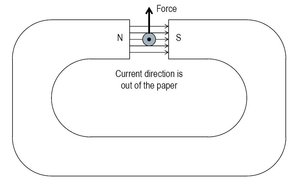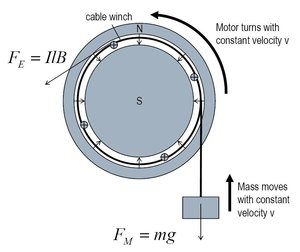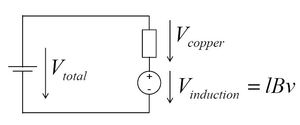The DC Motor

IntroductionFig. 1: Lorenz Force.

The electric motor is the primary means of converting electrical energy into mechanical energy. Millions of devices require electric motors to operate. They are found in everything, washing machines, electric screwdrivers, children's toys, and some of the new and very expensive cars. [1,2] Electric motors drive them all. Furthermore, the electric motor has been put to work for quite a long time, ever since its invention in the 1830s. As with most great inventions, there is some debate as to who invented the electric motor. Settling that debate is far beyond the scope of this paper, but I would be remiss if I did not give credit to some of the most famous people who helped develop the electric motor. Therefore I would direct the interested reader to the search engine of their choice where a few clicks will yield them piles of information on Allessandro Volta, Hans Christian Oersted, Andre-Marie Ampere, Michael Faraday, and many more. Just be careful that the history of the electric motor is very much dependent on the nationality of the historian. History aside, this article gives a brief high-level description of the electric motor, following closely the presentation in Hughes, which does not require a Ph.D. in physics to understand, some high school physics should be more than enough. 

The Electric Motor (DC)

The Lorenz Force is a well-known relation, which states that any current-carrying wire that passes through a magnetic field will feel a force due to that field. Fig. 1 diagrams a scenario in which the Lorenz Force is present and gives the formal definition

F = I (l × B)Fig. 2: Round Motor.

Without spending too much time on the equation it is worth noting that the Lorenz force is maximized when the electric current and magnetic fields are perpendicular. In this case the Lorenz force simplifies to the multiplication of the field strength B, the current magnitude I, and the length of the wire subjected to that field l. For the rest of this article we shall assume that some clever engineer has designed our electric motor to operate in this regime.

Fig. 2 depicts our setup for a simple electric motor, wherein a strong magnetic field is set up between an air gap. Current-carrying wires are placed in the gap and are attached to a winch, which is in turn connected to a mass via a cable. If we assume that the motor is in steady state (not moving), then the electromagnetic force on the wire FE must perfectly balance the mechanical force on the wire FM. This gives

 FM = FE → I = mglB

This tells us the total amount of current required to keep the system in equilibrium. Notice that every wire adds to the electric force, we lump the four wires into one equivalent wire to keep things simple. While it is the current that directly translates to force, a voltage is needed to set up that current. If our wire has a resistance R then from Ohm's law we know that the voltage needed to establish a given current is V = IR. We can calculate the strength of our electric motor by determining the power needed to hold a known mass steady. The electrical power input into the system is then

Pcopper = I2R

if we assume our magnetic field is set up with a permanent magnet then no other power is input into the system.Fig. 3: Simple electrical circuit model for a DC motor.

More interesting, however, is a motor that is moving. Let us now assume that we use enough power to lift the mass against the force of gravity. For simplicity let us assume that as a result of our efforts the wire moves to the left (Fig. 2) at a constant velocity and the mass rises at a similar constant velocity. In the case where the wire-mass system is moving at a constant velocity we know from Newton's first law that the forces must be equal and opposite, balancing each other. [4,5] Even still, our motor is still doing work and outputting power (so as to raise the block). The mechanical output power of our system is given as P = F v. This power must come from somewhere and is not captured by the resistive loss in the wire. Faraday's law states that moving a wire through a magnetic field will induce a voltage on that wire. As we drive current across this induced voltage we remove electrical power from the system (P = I V). This electrical power must balance the mechanical power transferred to the mass. Since the total input power is the sum of the copper loss and the mechanical power

Ptotal = Pcopper + Pmechanical

and the mechanical power must equal the electromagnetic force (the force of gravity when the system is not accelerating) we can solve for the steady-state velocity for a given input voltage and current:

Vtotal I = I2 R + I l B v

We can also solve for the induced voltage, by subtracting the copper losses from the total power

Vinduction = Vtotal - I R = l B v

which is Faraday's induction law. This simple solution can be reinterpreted as the simple electrical circuit depicted in Fig. 3. Electrically, the motor looks like the copper resistance of the wire in series with a velocity-dependent voltage source. As the speed of the motor increases so does this voltage. This model properly captures most of the important behavior of an electric motor. When the motor is not moving no mechanical power is transferred, and all of the input power is burned in the resistive loss of the wire. As the speed of the motor increases, so too does the power transferred to the load. Indeed at some high velocities the internally generated voltage is higher than the input voltage. In this case current flows in the reverse direction; our motor has become a generator, which converts mechanical power into electrical power.

Conclusion

This article developed a very basic linear motor model based on the discussion in Hughes.  It gives an intuitive explanation of both motor and generator operation furthermore it can be easily extended from a linear to a rotary motor, since force applied around an axis is torque. Finally, we should thank all of the engineers and physicists who have improved both electric motors and generators over the past 180 years, and have thus made the world a much better place.

© Martin Kramer. The author grants permission to copy, distribute and display this work in unaltered form, with attribution to the author, for noncommercial purposes only. All other rights, including commercial rights, are reserved to the author.

References

 T. Veltman, "Diesel-Electric Hybrids," Physics 240, Stanford University, Fall 2010.

 Y. Zhong, "Outlook of Electric Car Batteries," Physics 240, Stanford University, Fall 2011.

 A. Hughes, Electric Motors and Drives: Fundamentals, Types and Application, 3rd Ed. (Newnes, 2005).

 R. P. Feynman, R. B. Leighton and M. Sands, The Feynman Lectures on Physics (Basic Books, 2011).

 I. Newton et al., The Principia: Mathematical Principles of Natural Philosophy (U. California Press, 1999).